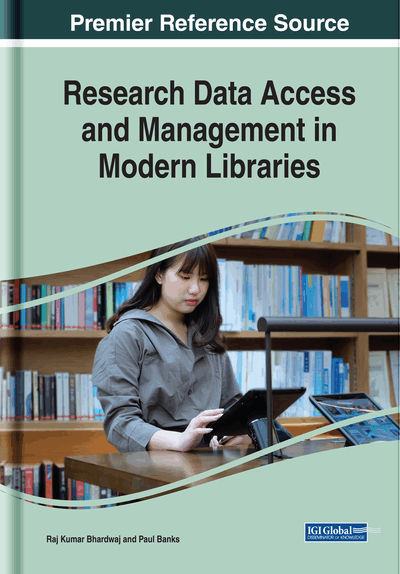# Research Data Analysis Using EViews: An Empirical Example of Modeling Volatility

Erginbay Uğurlu (Istanbul Aydın University, Turkey)
DOI: 10.4018/978-1-5225-8437-7.ch014
Available
\$37.50
No Current Special Offers

## Abstract

The aim of this chapter is to provide a detailed empirical example of autoregressive conditional heteroskedasticity (ARCH) model and selected generalized ARCH models. Before the ARCH/GARCH models are estimated, several calculations and tests should be done. The mean model is determined using the autocorrelation function and partial autocorrelation function and also the unit root test. The existence of ARCH effect is tested using ARCH-LM test. After these steps are done, then ARCH/GARCH models can be estimated. All these theoretical aspects are applied to Sofia Stock Indexes (SOFIX) using EViews 9 software package. The windows and output of EViews are presented. To show the output's academic writing format researchers' outputs are presented in a table.
Chapter Preview
Top

## Background Of Garch Models

Whereas heteroskedasticity is associated with models for cross-section data in textbooks, autoregressive conditional heteroskedasticity (ARCH) can be found in time-series models. Volatility is a vital concept for financial series. It is used in portfolio optimization, risk management, and asset pricing. The main problem of this kind of data is the impossibility of modeling using a linear model because this kind of data includes leptokurtosis, volatility clustering, long memory, volatility smile, and leverage effects. Also, one of the assumptions of linear models, which is homoscedasticity is not appropriate when using financial data (Floros 2008:35). In order to model volatility, Engle (1982) developed the Autoregressive Conditional Heteroscedastic (ARCH) models. Engle observed that large and small errors tend to occur in clusters, and then formulated it as follows: information from the recent past might influence the conditional disturbance variance. After Engle, Bollerslev (1986) extended ARCH to Generalized Autoregressive Conditional Heteroscedastic (GARCH) model. Bollerslev’s improvement is to add the forecasted variance from the last period (the GARCH term) to the volatility of the past (ARCH term). Nelson (1991) proposed the Exponential GARCH (EGARCH) model an EGARCH model that is often employed to capture the asymmetric effect of innovations on volatility. It is not the last GARCH type model, but in this chapter, we investigate only these three models.

### ARCH Model

ARCH models based on the variance of the error term at time t depend on the realized values of the squared error terms in previous time periods. The model is specified as:

(1)(2)

This model is referred to as ARCH(q), where q refers to the order of the lagged squared returns included in the model. If we use the ARCH(1) model it becomes

(3)

Conditional variance is presented byin the equation. Conditional variance must always be strictly positive to have meaningful variance at any point in time. To ensure that the conditional variance is a strictly positive coefficient in the equation must beand.

## Complete Chapter List

Search this Book:
Reset